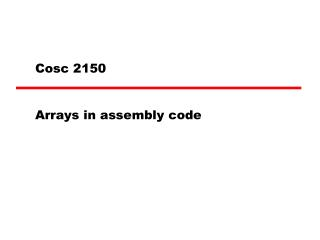# Cosc 2150 - PowerPoint PPT PresentationDownload PresentationCosc 2150

Cosc 2150
Download Presentation## Cosc 2150

- - - - - - - - - - - - - - - - - - - - - - - - - - - E N D - - - - - - - - - - - - - - - - - - - - - - - - - - -
##### Presentation Transcript

1. Cosc 2150 Arrays in assembly code

2. Variables and addresses • Uncompiled ld [a], %r1 addcc %r1, 2, %r3 • ARC has three addressing modes • immediate , direct, and indexed • In the following code segment. • compiled load , %r1 addcc %r1, 2, %r3 direct immediate

3. Variables and addresses (2) • Direct addressing • Like IAS, ARC loads the value at a memory location • So when, load [a], %r1 is “compiled”, a symbol table is built. A is located at memory location 2064 • Given us, the final code with load , %r1 • The [] tells us this is a direct addressing mode. • So given the following variables and memory a: 5 2064 b: 6 2068 c: 7 2072 d: 8 2076 e: 9 2080 • We can find each variable in a sequence “down” memory. • So load , %r1 ! load c’s value into %r1

4. Arrays vs variables • Using the following table • variable c points to memory location 2072 (direct) • arr points to memory location 2072, but we used index addressing. • the arr’s base address is 2064 plus 8, given us the address of 2072

5. Array addressing • ElementAddress = Base + (Index – Start)*size • Base = base address of the array • Start = array [-10..10] of integer • The start would be –10 • Note, In c/c++ starting index is normally 0 • Index = element in the array you want • array, index =5 • Size = size of individual element in bytes

6. Example • array [-10..10] of integer; array = 2; • EA = array + (5 – -10)*4 = array + 60 • add %r0, 5, %r1 ! load index • sub %r1, -10, %r1 ! subtract start • sll %r1, 2, %r1 ! multi by size =4 • add %r0, 2, %r2 ! load 2 • st %r2, array + %r1 !store 2, in array

7. C/C++, normally starts arrays at 0, • so the formula can be simplified to • EA = array + (index)*size • In ARC, the size will always be 4, so • EA = array + index*4

8. Visual example of an array in memory

9. int c; for (I=0; I<5; ++I) { c[I] = I+1; } Assembly shown in simulator .begin .org 2048 ! for (I=0; I<5; main: ld [I], %r2 ! load I into register 2 top: subcc %r2, 5, %r0 bpos done ! figure out array address in memory sll %r2, 2, %r1 !multi index by 4 ! Need I+1 add %r2 ,1, %r3 ! add I +1 ! store I +1 in c[I] st %r3, %r1 + c ! Increment I=I +1 add %r2, 1, %r2 st %r2, [I] ba top done: halt I: 0 c: 0 0 0 0 0 .end Example 1

10. Example 2 int a ={1,2,3,4,5}; int b ={0,0,0,0,0}; int I; for (I=0; I <5; ++I) { b[I] = a[I] + 2; } .begin .org 2048 ! for (I=0; I<5; main: ld [I], %r2 ! load I into register 2 top: subcc %r2, 5, %r0 bpos done ! figure out array address in memory sll %r2, 2, %r1 !multi index by 4 ! load a[I] and add 2 ld %r1+a, %r3 add %r3, 2, %r3 ! store a[I] + 2 in b[I] st %r3, %r1 + b ! I=I +1 add %r2 ,1, %r2 !add I +1 st %r2, [I] ba top done: halt I: 0 a: 1 2 3 4 5 b: 0 0 0 0 0 .end

11. Example 3 int a ={1,2,3,4,5}; int b ={5,4,3,2,1}; int I; for (I=0; I <5; ++I) { a[I] = a[I] + b[I]; } • again shown in simulator

12. .begin .org 2048 ! for (I=0; I<5; main: ld [I], %r4 ! load I into register 4 top: subcc %r4, 5, %r0 bpos done sll %r4,2, %r1 ! load a[I] into register 2 ld %r1+a, %r2 ! load b[I] into register 3 ld %r1+b, %r3 add %r2, %r3, %r5 ! store a[I] + b[I] in a[I] st %r5, %r1 +a add %r4, 1, %r4 st %r4, [I] ba top done: halt I: 0 a: 1 2 3 4 5 b: 5 4 3 2 1 .end ARC code, example 3

13. Example 4 How would we write the following code a[i] = b[i-1];

14. Q A &